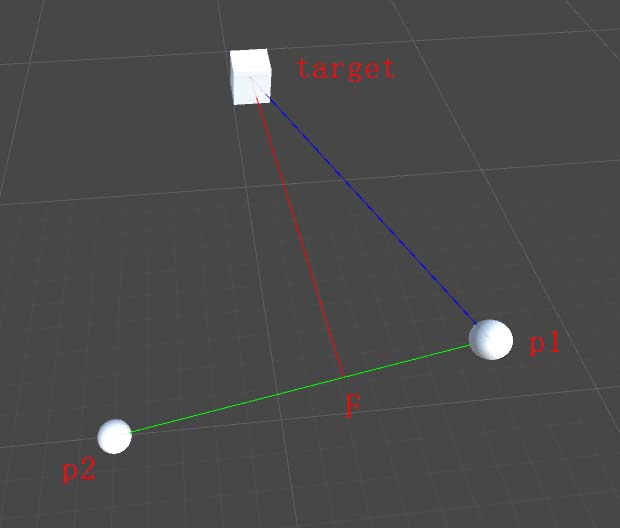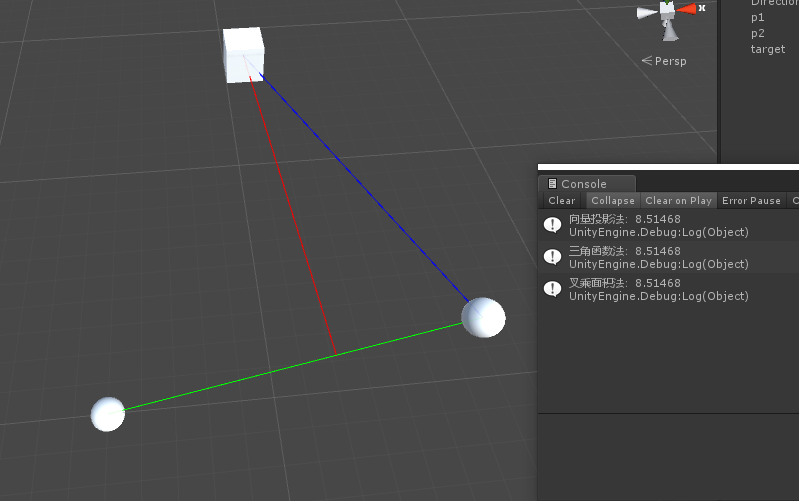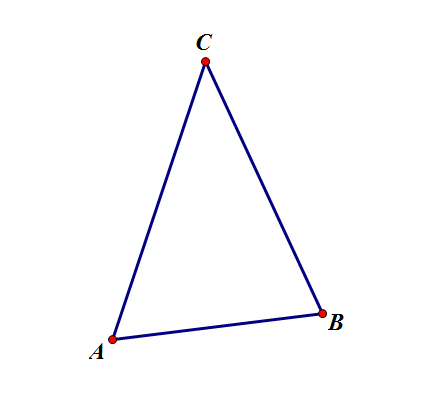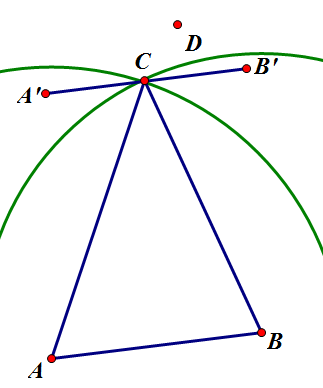• 【寒江雪】点到直线最短距离
千次阅读
2018-07-17 16:21:11

## 点到直线的最短距离

​ 根据直线的表示方式p = at + b，可以设一个点P(px,py)，任意一点A(x0,y0)到点P的距离可以根据两点间距离公式求出。

D^2 = (px-x0)^2+(py-y0)^2

= (axt+bx-x0)^2+(ayt+by-y0)^2

= (ax^2+ay^2)t^2 + 2{ax(bx-x0)+ay(by-x0)}t+(x0^2+y0^2)

​ 然后根据对表达式求二阶导数，可以证明该函数存在最小值。

​ 令一阶导数等于零可以求得取最小值时，t的取值。将t带入可以计算出最短距离的平方。最后算得

t = {ax(x0-bx)+ay(y0-by)}/(ax^2+ay^2)

## 点到线段的最短距离

​ 根据点到直线的距离的计算方式，把线段看成是直线，最后计算出t，然后根据t的值将t缩小到[0,1]之间。

if t < 0
than t = 0
if t > 1
than t = 1

​ 这样就可以计算点到线段的最短距离了。

更多相关内容
• 这两天做开发的时候，有个小伙伴问我怎么计算点到直线最短距离，看样子困扰了他挺久，所以借这个机会吧这个问题记录下来，让更多小伙伴能涨一下姿势。 先看图：已知条件如下，p1、p2、target三个，求红色线段的...

# 写在前面

这两天做开发的时候，有个小伙伴问我怎么计算点到直线的最短距离，看样子困扰了他挺久，所以借这个机会吧这个问题记录下来，让更多小伙伴能涨一下姿势。

先看图：已知条件如下，p1、p2、target三个点，求红色线段的距离。# 解题思路

## 思路1：向量投影法

这个方法核心问题是计算出target到p12向量上距离最短的点，然后通过Vector3.Distance方法计算得出最短距离。

那么如何找到这个点呢？这里，我用了向量投影。

1.先计算出target到p1的向量（蓝色线段）

2.再计算出p2到p1的向量（绿色线段）

3.通过Vector3.Project方法，计算出蓝色线段在绿色线段上的投影，这个投影就是p1F向量

4.将计算出来的投影向量再加上p1点的坐标，即使F点的坐标。

5.最后Vector3.Distance(target,F)即可计算出距离

代码如下：

    /// <summary>
/// 向量投影法
/// </summary>
public void ProjectFunc(Vector3 p1, Vector3 p2, Vector3 target)
{
//p1->p2的向量
Vector3 p1_2 = p2 - p1;
//p1->target向量
Vector3 p1_target = target - p1;
//计算投影p1->f
Vector3 p1f = Vector3.Project(p1_target, p1_2);
//加上p1坐标 然后计算距离
float distance = Vector3.Distance(target, p1f + p1);
Debug.Log("向量投影法：" + distance);
}

## 思路2：三角函数法

这个方法，就是通过Sin(夹角)*斜边长度计算直角边的距离

1.先计算出target到p1的向量（蓝色线段）

2.再计算出p2到p1的向量（绿色线段）

3.计算前面两个向量的夹角Vector3.Angle(p1_2, p1_target);

4.获取斜边长度（蓝色线段）

5.通过Sin函数*斜边长度计算出距离

代码如下：

    /// <summary>
/// 三角函数
/// </summary>
public void TriangleFunc(Vector3 p1, Vector3 p2, Vector3 target)
{
//p1->p2的向量
Vector3 p1_2 = p2 - p1;
//p1->target向量
Vector3 p1_target = target - p1;
//前面两个向量的夹角
float angle = Vector3.Angle(p1_2, p1_target);
//获取斜边的长度
float distance_p1_target = p1_target.magnitude;
//Sin函数计算距离
float distance = Mathf.Sin(angle * Mathf.Deg2Rad) * distance_p1_target;
Debug.Log("三角函数法：" + distance);
}

## 思路3：面积法

这个方法主要是通过平行四边形面积公式来计算出该距离 底 * 高 = 面积

现在我们知道了一条底边的长度（绿色线段），还差面积不知道

这里通过向量叉乘，获取叉乘结果的模就可以（叉乘出来的结果是个向量，向量的长度即为两个叉乘向量所形成的平行四边形的面积）

1.先计算出target到p1的向量（蓝色线段）

2.再计算出p2到p1的向量（绿色线段）

3.两个向量叉乘，获取的结果取长度，即为平行四边形的面积

4.然后 高 = 面积 / 底 得出距离

代码如下：

    /// <summary>
/// 叉乘面积
/// </summary>
public void CrossAreaFunc(Vector3 p1, Vector3 p2, Vector3 target)
{
//p1->p2的向量
Vector3 p1_2 = p2 - p1;
//p1->target向量
Vector3 p1_target = target - p1;
//两个向量叉乘结果取长度 用做面积
float area = Vector3.Cross(p1_2, p1_target).magnitude;
//面积/底边计算出高度
float distance = area / p1_2.magnitude;
Debug.Log("叉乘面积法：" + distance);
}

# 最后

这里展示了三种获取点到线的最短距离的方式方法，运行结果也都一致，这里做一个记录，希望能帮助到需要帮助的小伙伴。展开全文unity unity3d 数学
• 假设这个区域存在一点D，显然有点D到直线AB的距离 大于 C到直线距离 即△DAB的最大高 大于 △CAB的最大高，与前提“拥有最大高的三角形是ABC”矛盾 得证 在AB下侧，那两个圆的交点和C是关于AB对称的，道理...

Ofelia is chased by her evil stepfather, and now she finds herself lost in a labyrinth. She needs your help to run away from her tragic family.

There's a huge metal door standing in front of the exit of the labyrinth. There are n dots on the metal door. Pan (the god of the labyrinth) asks Ofelia to find out a triangle which has the largest height. The triangle's three vertexes must be the dots on the door, and its area must be positive. Ofelia should tell Pan the triangle's height so Pan will let Ofelia go.

#### Input

There are multiple cases (About 100 cases). For each case, the first line contains an integer n (3<=n<=500). In the next n lines, each line contains two real number x[i]y[i] (0<=x[i], y[i]<=10000) which indicates each dot's coordinate. There is no two dots in the same coordinate. The answers are strictly greater than 0.

#### Output

For each test case, output one line with the maximum triangle height. Any solution with a relative or absolute error of at most 1e-6 will be accepted.

#### Sample Input

6
0.000 4.000
2.000 4.000
1.000 0.000
1.000 8.000
0.900 7.000
1.100 7.000
7
6967.54555 3457.71200
3.52325 1273.85912
7755.35733 9812.97643
753.00303 2124.70937
7896.71246 8877.78054
5832.77264 5213.70478
4629.38110 8159.01498



#### Sample Output

7.00000
8940.96643

题意：给出一系列的坐标，要求出这些坐标中组成的三角形中最大的高是多少
思路：
假设拥有最大高的三角形是ABC，如下图结合此图我们可以看出最大高是点C到直线AB，在这种情况下，下列两个假设必然有一个成立1.点C是所有点中距离点A最远的2.点C是所有点中距离点B最远的
证明：（这里先证明在AB上侧成立的情况）首先过点C做直线A'B'平行于AB，再分别以点A、点B为圆心，AC、BC长为半径做圆假设上述两条都不成立，则显然只能在直线A'B'以上且在圆A、B外区域的点才符合假设这个区域存在一点D，显然有点D到直线AB的距离 大于 点C到直线的距离即△DAB的最大高 大于 △CAB的最大高，与前提“拥有最大高的三角形是ABC”矛盾得证
在AB下侧，那两个圆的交点和点C是关于AB对称的，道理也一样，就不赘述了因此我们可以枚举点A，先求得距离他最远的点C，再枚举点B就可以了

以上解释来自：http://blog.csdn.net/accelerator_916852/article/details/20394799

#include <stdio.h>
#include <string.h>
#include <math.h>
#include <algorithm>
using namespace std;

struct point
{
double x,y;
}p;

double dist(point a,point b,point c)
{
if(b.x!=a.x)
{
double k=(b.y-a.y)/(b.x-a.x);
return fabs((c.y-a.y)-k*(c.x-a.x))/sqrt(1+k*k);
}
else
return fabs(c.x-a.x);
}

double len(point a,point b)
{
return sqrt((a.x-b.x)*(a.x-b.x)+(a.y-b.y)*(a.y-b.y));
}

int main()
{
int n,i,j,f;
double maxn,t,ans;
while(~scanf("%d",&n))
{
ans = 0;
for(i = 1;i<=n;i++)
scanf("%lf%lf",&p[i].x,&p[i].y);
for(i = 1;i<=n;i++)
{
maxn = 0;
for(j = 1;j<=n;j++)
{
if(i==j)
continue;
t = len(p[i],p[j]);
if(t>maxn)
{
maxn = t;
f = j;
}
}
for(j = 1;j<=n;j++)
{
if(j == i || j == f)
continue;
ans = max(dist(p[i],p[j],p[f]),ans);
ans = max(dist(p[i],p[f],p[j]),ans);
ans = max(dist(p[f],p[j],p[i]),ans);
}
}
printf("%.6f\n",ans);
}

return 0;
}

展开全文• 求空间中一个的坐标，这个坐标n多个直线距离最短，n已知，直线方程已知优化 算法
• 一、根据两直线方程 已知直线上两为：(x1,x2),(y1,y2); 设方程为：Ax+By+C=0; 1. 求斜率：k=(y2-y1)/(x2-x1)；...1. (x0,y0)到直线距离公式为： d=abs(Ax0+By0+C)/sqrt(A*A+B*B); 2. 设垂足为p,则：

## 一、根据两点求直线方程

已知直线上两点为：(x1,x2),(y1,y2);

设方程为：Ax+By+C=0;

1. 求斜率：k=(y2-y1)/(x2-x1)；

2. 直线方程为： y-y1=k(x-x1)；

换算得：kx-y+y1-kx1=0,即：

A=k
B=-1
C=y1-kx1=y1-(y2-y1)/(x2-x1)*x1

## 二、求距离和垂足公式

1. 点(x0,y0)到直线的距离公式为：

d=abs(Ax0+By0+C)/sqrt(A*A+B*B);

2. 设垂足为p,则：

p.x=(B*B*x0-A*B*y0-A*C)/(A*A+B*B)
p.y=(A*A*y0-A*B*x0-B*C)/(A*A+B*B) 

## 三、 代码

输入点和线段，返回距离和垂足1 function getDistanceP2L(point,line){
2
3 var x1=line.startPoint.x;
4 var y1=line.startPoint.y;
5 var x2=line.endPoint.x;
6 var y2=line.endPoint.y;
7 var x0=point.x;
8 var y0=point.y;
9
10 var k=x1==x2?10000:(y2-y1)/(x2-x1);//当x1=x2时，给斜率设一个较大值10000
11 var a=k;
12 var b=-1;
13 var c=y1-k*x1;
14
15 var d=Math.abs(a*x0+b*y0+c)/Math.sqrt(a*a+b*b);
16
17 var px=(b*b*x0-a*b*y0-a*c)/(a*a+b*b);
18 var py=(a*a*y0-a*b*x0-b*c)/(a*a+b*b);
19 var p=new Point(px,py);
20
21 return [d,p];
22 }展开全文vc++
• 转自：http://hi.baidu.com/javalovers/item/74b6e60f9debaae6f45ba657// 点到直线最短距离的判断 (x0,y0) 由两组成的线段(x1,y1) ,( x2,y2 )privatedoublepointToLine(intx1,inty1,intx2,inty2,intx0,inty0...
• c语言已知两坐标，求另一点穿过这两直线最短距离。以下文字资料是由(历史新知网www.lishixinzhi.com)小编为大家搜集整理后发布的内容，让我们赶快一起来看一下吧！c语言已知两坐标，求另一点穿过这两...
• 这个计算点到线段最短距离算法是先计算线段上距离最近的，然后求出 2...在计算用户当前位置某条公路的最短距离等实际情况中，可以先用这个算法，然后根据结果坐标使用 CLLocation 类的函数计算实际距离 VC6工程
• 记录下 java jts 求两个空间几何图形间最短距离，及最短距离间的两个坐标. 如：求一个点到一条直线的垂直坐标
• 一、根据两直线方程 已知直线上两为：(x1,x2),(y1,y2); 设方程为：Ax+By+C=0; 1. 求斜率：k=(y2-y1)/(x2-x1)； 2. 直线方程为： y-y1=k(x-x1)； 换算得：kx-y+y1-kx1=0,即： A=k B=-1 C=y1-kx1=y1-...
• p 到直线 ab 的距离：|p x ab| / |ab| |p x ab|是 p 和 ab 形成的四边面的面积，那么除以 底边|ab| 就是高，即 p ab 的距离 根据向量表示 向量AP可以表示为（Px-Ax，Py-Ay，0） 向量AB（Bx-Ax，By-Ay...
• 今天小编就为大家分享一篇Python求平面内点到直线距离的实现，具有很好的参考价值，希望对大家有所帮助。一起跟随小编过来看看吧
• 点到直线距离： struct Node { int x,y; }; double D(Node a,Node b,Node c)//计算点到直线距离 { double d=(fabs((b.y-a.y)*c.x+(a.x-b.x)*c.y+((b.x*a.y)-(a.x*b.y))))/(sqrt(pow(b.y-a.y,2)+pow(a.x-b.x,2)))...
• 而且基本适用于所有点到直线距离的情况。 这次教给大家一个用几何的方法计算A线BC的距离。代码如下： public double GetPointToLineDistance(XYZ aPoint, Line l) { //先分别得到的坐标 XYZc# 软件开发
• 点到线段最短距离的运算与点到直线最短距离的运算二者之间存在一定的差别，即求点到线段最短距离时需要考虑参考在沿线段方向的投影是否在线段上，若在线段上才可采用点到直线距离公式
• 泛函 泛函是函数的函数，定义域是函数...两之间直线最短 可以看出，初等数学为高等数学的推导提供了依据，高等数学反过来又能证明初等数学。 参考视频： 为什么两之间直线段距离最短？谈谈泛函与变分法...泛函
• private double pointToLine(int x1, int y1, int x2, int y2, int x0,int y0) {double space = 0;double a, b, c;a = lineSpace(x1, y1, x2, y2);// 线段的长度b = lineSpace(x1,...// (x1,y1)到点的距离c = lineSp...
• //计算点到直线距离 double point2LineDistance(cv::Point2d point, cv::Vec4f lineParam) { cv::Point2d qIpoint; cv::Vec4f lineVert; lineVert = lineParam; lineVert = -lineParam; lineVert...c++ 计算机视觉
• 博客转载自：http://blog.sina.com.cn/s/blog_5d5c80840101bnhw.html点到线段最短距离的运算与点到直线最短距离的运算二者之间存在一定的差别，即求点到线段最短距离时需要考虑参考在沿线段方向的投影是否在线...
• 矢量法推导： 求P线段AB的最短距离。分成以下三种情况(a),(b),(c)。所以可以先根据计算出r的值，进而对应计算A B C 和 P之间的距离即可。特殊情况：当P在线段AB上：计算出来r仍然是 1&gt;r&gt...矢量算法
• 只回答定点折线的最短距离的求法。一般由目测，即可看出哪一段折线是离定点最近的折线(例如你画的那个，显然DE就是离定点最近的折线段)，如看不出多算几段也可以，例如可以算每段折线离定点最近的，则所有线段中...
• 直线的信息可以以两个端点的形式给出，也可以以一个直线上的直线的方向向量给出。本文中假设这两条直线不...求两直线最短距离直线l0我们可以用方程表示为：  （1） 直线段l1我们也可以用方程表示为：c++编程 最小二乘法 计算机图形学
• 一种点到线段的最短距离的方法 下面介绍点到线段的最短距离的方法： 图 1-6点到线段的最短距离 图1-6描述了一种计算D线段AC的最短距离的计算方法。直线方程由经过两A (x1,y1) 和 C (x2,y2)确定： B = A ...
• 已知两(x1, y1) 和 (x2, y2)，求某(x, y) 确定的直线距离 代码 // 获取点到直线距离 function getPointToLineDistance(list, point){ let [[x1, y1], [x2, y2]] = list; let [x, y] = point; if(x1...Math.sqrt Math.abs
• d1到直线d2 d3的距离 并画线 范例： [d dl] find dl [2 1] [8 3] [4 7] ; 则 dl [6 5]
• } } // 计算两条直线之间的距离 // E是直线AB上一点，F是直线CD上一点，线段EF是直线AB到直线CD距离最短的线段 // 返回值为线段EF的长度 template float MyLineToLine(const T& A, const T &B, const T &C, const T...c++
• function [xy,distance,t_a] = distance2curve(curvexy,mapxy,interpmethod) % distance2curve: minimum distance from a point to a general curvilinear n-dimensional arc % usage: [xy,distance,t] = distance2......